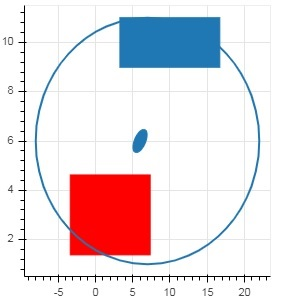# Bokeh - Rectangle, Oval and Polygon

It is possible to render rectangle, ellipse and polygons in a Bokeh figure. The rect() method of Figure class adds a rectangle glyph based on x and y coordinates of center, width and height. The square() method on the other hand has size parameter to decide dimensions.

The ellipse() and oval() methods adds an ellipse and oval glyph. They use similar signature to that of rect() having x, y,w and h parameters. Additionally, angle parameter determines rotation from horizontal.

### Example

Following code shows use of different shape glyph methods

```from bokeh.plotting import figure, output_file, show
fig = figure(plot_width = 300, plot_height = 300)
fig.rect(x = 10,y = 10,width = 100, height = 50, width_units = 'screen', height_units = 'screen')
fig.square(x = 2,y = 3,size = 80, color = 'red')
fig.ellipse(x = 7,y = 6, width = 30, height = 10, fill_color = None, line_width = 2)
fig.oval(x = 6,y = 6,width = 2, height = 1, angle = -0.4)
show(fig)
```

### Output